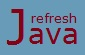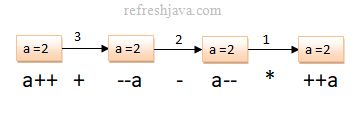# Unary Operators in Java with Examples

Unary simply means one, so unary operators are the operators which operates on only one operand. These operators are also quite used by java programmers. The table below shows the list of all unary operators in java. For this table let's assume we have an integer variable `a` and a boolean variable `isValid` :

Operator Name Example Description
+ Unary plus operator +a, +5 Indicates positive value
- Unary minus operator -a, -3 Negates an expression
++ Increment operator a++, ++a Increments value of `a` by 1
-- Decrement operator a--, --a Decrements value of `a` by 1
! Logical complement operator !`isValid` Inverts the value of a boolean

## Java unary plus and minus operator

Unary plus operator indicates that a number is positive. By default numbers are positive, the number 2 and +2 are same, so it doesn't matter whether you have used or not used this operator with a variable/value. The unary minus operator negates a variable/value, that means this operator makes a positive number as negative and a negative number as positive.

The operand in an unary plus and minus operator can be a constant value, a variable, an expression or a function call returning a compatible value.

``` `int` a = +10, b = -20; ` // operand can be a constant value`
`int` c = -a;` // operand can be a variable`
`int` d = -(a+b);` // operand can be an expression`
`int` e = -sum(a,b);` // operand can be a method(sum) call`
```

## Java logical complement operator

The logical complement operator(!) inverts the boolean value, if the boolean value is `true`, it becomes `false` and if it is `false`, it becomes `true`. The operand in logical complement operator must be a boolean value, a boolean variable, a boolean expression or a method call returning a boolean value. The operand can not be a constant value.

``` `boolean` b = !`true`; ` // operand can be a boolean value // b = false`
`boolean` c = !b;` // operand can be a boolean variable // c = true`
`boolean` d = !(10 > 5);` // operand can be an expression // d = false`
`boolean` e = !isValid();` // operand can be a boolean method(isValid) call`
`boolean f = !10;`` // Incorrect, operand can't be a constant value`
```

## Increment and decrement operators in Java

The unary increment(++) operator increments the value of a variable by `1` while unary decrement(--) operator decrements the value of a variable by `1`. For example `a++` increases the value `a` by `1` and is same as `a = a + 1`, similarly `a--` decreases the value of `a` by `1` and is same as `a = a - 1`. Both these operators can be used with a variable only, not with any constant value, expression or method calls.

``` `int` a = 2, b = 3;
a++; ` // is same as ` a = a + 1;
a--; ` // is same as ` a = a - 1;
`// Following is incorrect declaration`
`int d = 2++;`` // increment/decrement can't be used with constant value`
`d = (a+b)++;`` // increment/decrement can't be used with expression`
`d = sum(a,b)++;`` // increment/decrement can't be used with method`
```

The unary increment/decrement operator can be applied before(prefix) or after(postfix) the variable name. Both versions, `++a` and `a++` will increase the value of `a` by one. The next section explains more detail about both the operators.

### Pre and post increment and decrement operators in Java

If the unary ++ operator is used before the variable name, it is called pre-increment operator while if it is used after the variable name, it is called post increment operator. Similarly if the unary -- operator is used before the variable name, it is called pre-decrement operator while if it is used after the variable name, it is called post decrement operator.

For example in code `++a;` the operator `++` is a pre-increment operator while in code `a++;` the operator `++` is post increment operator, the same applies with `--` operator as well.

In pre increment/decrement, the value of the variable is incremented/decremented first then it is assigned or evaluated, that is why it is called pre-increment/decrement. While in post increment/decrement the current value of the variable is assigned or evaluated, after that the increment/decrement happens, that is why it is called post increment/decrement. Let's understand this by the example below :

``` `int` a = 2, b = 3, c = 4, d = 5;
`int` p = a++; `// p = 2; first assignment happens then the increment`
p = a; `// a = 3, p = 3; ``// a was increased in previous line`
`int` q = ++b; `// q = 4; first increment happens then the assignment`
`int` r = c--; `// r = 4, first assignment happens then the decrement`
r = c; `// c = 3, r = 3; ``// c was decreased in previous line`
`int` s = --d; `// s = 4; first decrement happens then the assignment`
```

So in general, in case of pre increment/decrement operation, use the incremented/decremented value at the place of variable while in post increment/decrement, use the current value at the place of variable. Let's understand this with an expression to make it more clear.

``` `int` a = 2;
`int` p = a++ + --a - a-- * ++a;
```

To understand it clearly let's see step by step how this expression is evaluated. Just remember in java an expression is evaluated from left to right.

```  a++ + --a - a-- * ++a; `// original expression`
2 + --a - a-- * ++a; `// current value of a is 2, so a++ is replaced with 2, then the increment happens`
2 + 2 - a-- * ++a; `// at --a, the value of a was 3(was incremented with first a++) but pre decremented as 2`
2 + 2 - 2 * ++a; `// current value of a is 2 so a-- is replaced with 2, then it will be decremented`
2 + 2 - 2 * 2; `// Now current value of a is 1 so ++a will be replaced as 2 as it's a pre increment.`
2 + 2 - 4; `// first multiplication will happen as it has higher precedence over + and -`
0 `// So final value will be 0 which will be assigned in p`
```

The diagram below demonstrates how the value of variable `a` is evaluated.### What is prefix and postfix operators in Java ?

The pre increment/decrement operator is also known as prefix operator in java while the post increment/decrement operator is also known as postfix operator in java.

### What is the difference between pre increment and post increment ?

As mentioned above, in pre increment the value of the variable is increment first then it used or assigned in an expression while in post increment the current value of the variable is used or assigned in an expression then it is incremented.

### Java Program of Unary Operators

```` class` UnaryOperator {
`public static void` main(String[] args) {
`int` result = +5;
System.out.println(`"result = "`+result);
result--;
System.out.println(`"result = "`+result);
result++;
System.out.println(`"result = "`+result);
result = -result;
System.out.println(`"result = "`+result);
`int` i = 5;
i++;
System.out.println(`"i = "`+i); `// prints 6`
++i;
System.out.println(`"i = "`+i); `// prints 7`
System.out.println(`"i = "`+ ++i);  `// prints 8`
System.out.println(`"i = "`+ i++);  `// prints 8`
System.out.println(`"i = "`+i); `// prints 9`
System.out.println(`"i = "`+ --i); `// prints 8`
`boolean` isValid = `false`;
System.out.println(`"isValid = "`+ !isValid);
}
} ```

Output:

result = 5
result = 4
result = 5
result = -5
i = 6
i = 7
i = 8
i = 8
i = 9
i = 8
isValid = true

The program below demonstrates the pre and post increment/decrement operator in java, you can evaluate the value of different expressions in this example by yourself first then match your result with the output to see if you have understood it properly.

### Java program for increment and decrement operators

```` class` IncrementDecrement {
`public static void` main(String[] args) {
`int` a = 2, result;
result = a++ + --a - a-- * ++a;
System.out.println(`"result = "`+result+`", a = "`+a);
result = ++a * --a + a-- - a++;
System.out.println(`"result = "`+result+`", a = "`+a);
result = --a * ++a / ++a - a++ + a--;
System.out.println(`"result = "`+result+`", a = "`+a);
}
} ```

Output:

result = 0, a = 2
result = 7, a = 2
result = 1, a = 3

### How many unary operators are there in Java ?

There are 5 unary operators in java, + - ++ -- and !.